﻿ Application of the Euler-Maclaurin Sum Formula to Obtain a Closed form Analytical Solution for Acoustical and Heat Diffusion in a Partially Bounded RegionPublications are Open
Access in this journal
Article Versions
Export Article
• Normal Style
• MLA Style
• APA Style
• Chicago Style
Research Article
Open Access Peer-reviewed

### Application of the Euler-Maclaurin Sum Formula to Obtain a Closed form Analytical Solution for Acoustical and Heat Diffusion in a Partially Bounded Region

Michael Panza
American Journal of Mechanical Engineering. 2020, 8(3), 111-121. DOI: 10.12691/ajme-8-3-3
Received September 04, 2020; Revised October 06, 2020; Accepted October 15, 2020

### Abstract

Green’s function in the Laplace transform domain for the sound wave field in an infinite area between two parallel reflecting planes may be represented as an infinite series of images caused by the planes. Based on a comparison of the wave equation and the diffusion equation, the Green’s function representation as an infinite series for diffusion in the same region is directly obtained. A closed form expression in space time for the diffusion problem is obtained by applying the Euler-Maclaurin sum formula to a modified diffusion form of the series in the Laplace transform domain and then inverting to the time domain. Using several sets of numerical values for system parameters applicable to acoustic diffusion in the region, numerical comparisons of the infinite series vs Euler-Maclaurin closed form representation of the Green function is presented. Comparison of the infinite series vs Euler-Maclaurin transient response to an exponential and constant input are presented for cases of acoustical noise diffusion and heat diffusion respectively. Transfer function comparisons are given for the diffusion models along with the use of the closed form representation in a model-based control scheme.

### 1. Introduction

The application of the Euler-Maclaurin sum formula to determine an accurate closed loop solution to the infinite series of acoustic images in a partially bounded three-dimensional region was presented in 1 and repeated in 2 for a two-dimensional region. The work in 1 and 2 develops a closed form Green’s function that can be used to analyze inputs that excite a broad band of mid to high frequencies which are typically dominant in audible noise. The closed form solution was shown to be very accurate for both transient response to an external input and steady state response to a broad band frequency input. Additionally, the work in 2 provided frequency domain results for both feed forward and feedback control schemes to meet a desired objective. The work in 3, 4 extended the idea of a closed form solution obtained from the Euler-Maclaurin formula to develop a finite state space model to represent the infinite series that results from systems described with a wave equation where there is at least one bounded dimension. In 3, a string under tension is the basis for applying the Euler-Maclaurin sum formula to the infinite series that results for fixed string boundaries. The closed loop Green function is then transferred to a closed expression for the system transfer function which in turn is transformed into a state space system with a finite number of states and terms with a time delay. The state model derived for the string wave equation is then applied to predict the broadband vibrations of a plate. In 4, a similar procedure to that used in 3 is applied to the closed form solution of the infinite acoustic space from 1. In 3, 4, several examples of inputs including transient exponentials and random like noise are used to demonstrate the accuracy of the finite state space model for output prediction, output control, and input estimation.

The acoustic diffusion partial differential equation (which has the same form as the heat diffusion partial differential equation) has been analyzed for three-dimensional acoustic spaces using a variety of techniques. The work in 5 uses a special finite difference method for the acoustic diffuse partial differential equation describing a well-proportioned three-dimensional room (room with similar dimensions) and compares it to the Sabin diffusion formula. Also, in 6 a special finite difference method is applied to analyze cubic shaped rooms of various sizes. The work in 7 uses a commercial acoustics finite element model for diffusion to analyze the diffuse sound field in a cubical domed worship space. Acoustic diffusion in a disproportioned three-dimensional room such as flat and long rooms has been analyzed in 8 using commercial acoustics software. The work in 9 uses an infinite ray tracing model to predict the diffusion sound field in disproportioned three-dimensional rooms.

In this paper, the goal is to determine a closed loop analytical expression to represent the acoustic or heat diffusion in a large area between two perfectly reflecting planes for acoustical noise and for a finite insulated slab for heat conduction. The boundary conditions for each case are identical in that an infinite x-y plane is considered with a finite section in the z direction with no energy flowing across the z boundaries. The method involves applying the Euler-Maclaurin sum formula to a modified Laplace transform representation of infinite series of acoustic images between the two reflecting planes that comprise the Green function for acoustic field in the region. The modification involves comparing the wave partial differential equation for which the infinite series is obtained with the diffusion partial differential equation and changing the parameter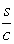in the series terms from the acoustic wave equation to the parameter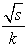for the diffusion equation. In these parameters, c is the speed of sound, k is the acoustic diffusivity, and s is the Laplace transform variable. The procedure is similar to that in 1 where the Euler-Maclaurin sum formula is applied to the infinite series in the Laplace transform domain and inversion provides a closed form solution in the time domain. The resulting closed form expression for the Green’s function may be used for acoustic, thermal, or any other diffusion application for the purpose of obtaining insight into system behavior, predictions for any type of input via convolution, or used in model based control methods.

### 2. Mathematical Model for Green’s Function

2.1. Series Solution of Diffusion Equation from Wave Equation

The basic concept is to compare the three dimensional wave and diffusion partial differential equations to show how the solution to acoustic wave problem between two perfecting parallel plates can be extended to the diffusion problem of noise between the two planes or to heat conduction in a slab with insulated surfaces. Figure 1 is a schematic of the three-dimensional space bounded on two sides by a plane with no energy flow through it and with the other two dimensions infinite.

• Figure 1. Schematic of a diametrical cross section of the partially bounded region

The acoustic wave equation and the thermal diffusion equation are given below where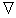is the Laplacian operator and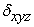is a Dirac delta function for three space dimensions.

For acoustic pressure p and propagation in a medium of sound speed c and point source input vA(t):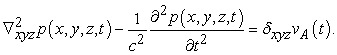(1)

For heat temperature T and diffusion in a medium of thermal diffusivity k and point source input vT(t):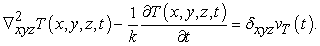(2)

For no energy flow at the parallel surfaces at z=0 and z=H, the modes across z are proportional to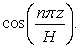This satisfies the boundary conditions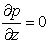andat these surfaces.

The partial differential equation for Green’s function for Eq. (1) and Eq. (2) differs only in the time derivative term. Defining Green’s function in the region between two perfecting parallel plates as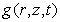where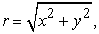the partial differential equations for Green’s function are given by replacing the right hand side of Eq. (1) and Eq. (2) by the Dirac delta functions δ(r-ro) and δ(z-zo) in the space domain and the Dirac delta function δ(t) in the time domain as given in 10.

For wave equation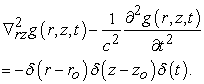(3)

For diffusion equation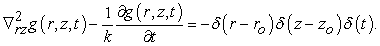(4)

Using the Laplace transform with respect to time t (t→s) ofand Eq. (3) and Eq. (4) are written as

For wave equation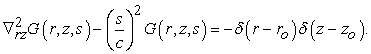(5)

For diffusion equation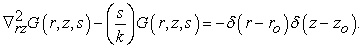(6)

Rewritingin the diffusion equations asEq. (5) and Eq. (6) have the same form given by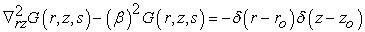(7)

where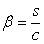for the wave equation and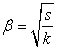for the diffusion equation.

Viewing Eq. (7) from an acoustic vantage point of Eq. (5), a solution can be expressed as an infinite sum of images proving acoustic rays from both sides of the parallel plates as given in 11. It is given by(8)

The work in 1 applied the Euler-Maclaurin sum formula to the sums in Eq. (8) to obtain an accurate approximate closed form solution for the acoustic wave region where2.2 Application of Euler-Maclaurin Sum Formula to the Diffusion Series

The form of the Euler-Maclaurin sum formula used is given in 1, 2, 3, 4 as(9)

where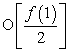is a remainder with O meaning and order “at most”.

Using ε for z+zo, z-zo, -z+zo, -z-zo, the inverse Laplace transform for the general sum term integral in Eq. (8) is determined in 1 to be given by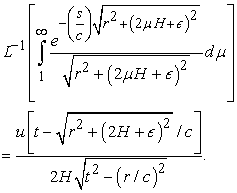(10)

The approximate closed form Green’s function for the acoustic wave region is then given by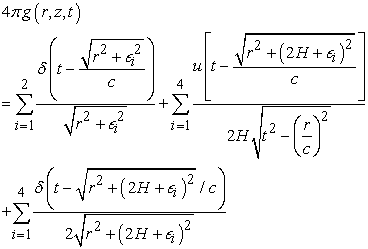(11)

In this paper we seek an approximate closed form solution for the diffusion region by applying the Euler-Maclaurin sum formula in Eq. (9) to the sums in Eq. (8) withThe series from Eq. (8) for diffusion is then given as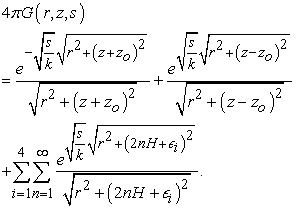(12)

The Euler-Maclaurin formula is applied to the infinite series in Eq. (12) to give(13)

The integral part of the Euler-Maclaurin sum in Eq. (13) is defined as I and expressed as(14)

Using the same procedure as that in 1, the inverse Laplace transform s → t in Eq. (14) withis given in 12 as(15)

where α in the numerator of L-1 cancels the α in the denominator of the integrand in Eq. (14).

In the time domain, the integral I from Eq. (14) becomes(16)

Factoring out the first part of the exponential gives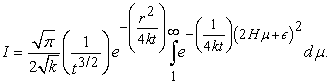(17)

Defining the integral in Eq. (17) asletting y=2Hμ+ε whereby dy=2H dμ,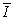is given by(18)

Now lettingwhereby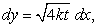the integral in Eq. (18) becomes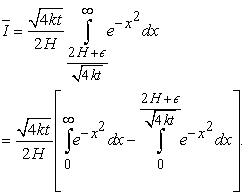(19)

Using the error function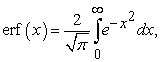Eq. (19) is given by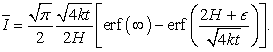(20)

The Euler-Maclaurin integral I in Eq. (17) is the product ofin Eq. (20) and the term. With erf(∞)=1, I is given by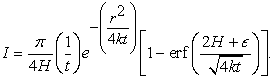(21)

Using the inverse Laplace Transform from Eq. (15) on the basic form of the non-integral terms in the Euler-Maclaurin sum of Eq. (13), the complete closed form expression for the Green’s function in the time domain is given as(22)

This may be simplified by using the case z=zo=0 which will be shown to be an accurate representation along the z direction. Eq. (22) becomes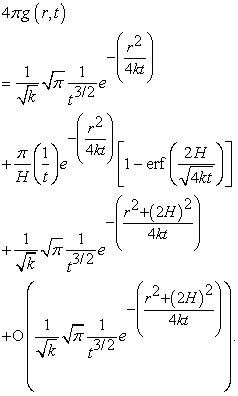(23)

The series form of the Green’s function in the time domain is obtained by taking the inverse Laplace of Eq. (8), which gives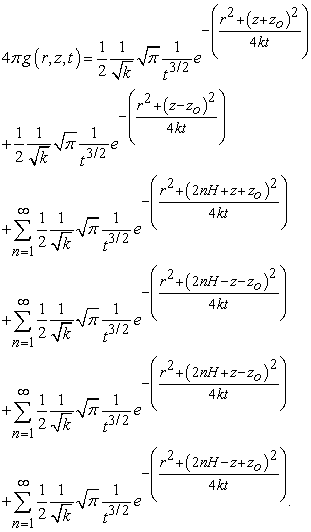(24)

The units of 4πg are 1/(m-sec) for Eq. (23) and Eq. (24).

### 3. Numerical Simulations for Green’s Function Comparison

For the application of acoustic diffusion, the Euler-Maclaurin closed form Green’s function solution of Eq. (23) is first compared to the Series Green’s function solution of Eq. (24) for several cases of H, r, and z. The value zo=0 is used for all cases. The value of the acoustic diffusivity k is calculated from the analytical method in 13 for a three-dimensional acoustic space of Volume V and boundaries surface area S . It is expressed as, where λ is the mean free path given byand c is the speed of sound.

For the acoustic space between two infinite reflecting planes of this paper, a cylindrical region of radius r and height H is used. For V=πr2H and S=2(πr2)+2πrH, the mean free path is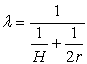and the acoustic diffusivity is given by(25)

Thus, the acoustic diffusivity k in the partial differential equation is a function of the radial location from the source location at r=0.

Numerical simulations for a wide range of parameters in the acoustic diffusion model are given for H=0.3 m to H=18 m and for r=.9 m to r=10.5 m which cover a practical range from tight acoustical enclosures to large industrial spaces. The work in 14 shows how a summation of images group model provides a means of estimating the internal sound level and noise reduction of a tight-fitting enclosure over a machine without having to estimate or measure the initial hard wall sound absorption. In 14, a prediction of the variance with distance from machine surface to enclosure surface is given. The results in 14 also show that the behavior of a standard large room diffuse field model does not provide this variance and is very sensitive to initial absorption conditions.

• Figure 2. Effect of location z relative to r for H=.6 m on the multi-reflective type terms in the four sums of Series form Eq. (24). z=0 (________), z=2.4 m. (- - - - - -), z=4.5 m (- . - . -. -. )

Figure 2 shows that the variation of the series solution from Eq. (24) with respect to vertical location z is small enough for times greater than about 0.05 seconds and thus z=0 may be used for comparisons with the closed form Euler-Maclaurin solution Eq. (23) derived with z=0. The figure gives the four sums from Eq. (24) which represent the reverberation between the planes.

The first two terms in the Series form of Eq. (24) represent the direct field from source to receiver location and are identical to the first two terms in the Euler-Maclaurin form in Eq. (23).

The remainder term O( ) in the Euler-Maclaurin Green function of Eq. (23) ranges from 0 to this upper order result, which is the same as the third term, given as 1/2 f(0). Figure 3 gives simulations for a couple of H, r sets to cover a range of parameters from a typical close fitting enclosure to a typical industrial space for the purpose of showing that the remainder term in the Euler-Maclaurin solution may be considered small enough to be neglected compared to the main integral term of Eq. (23). The figure also shows how the identical initial z=zo=0 direct field terms in both the Euler-Maclaurin and the series representations contribute to the total Green function. As expected, these direct field terms are prevalent during the initial times and then are dominated by the Euler-Maclaurin integral part as time increases.

• Figure 3. Comparison of Euler-Maclaurin closed form to Series form of total solution (direct + reverb), including components. (a) H=.3 m, r=.9 m, (b) H=3 m., r=6 m. First column: EM Eq. (23) (________) vs. Series Eq. (24) (- - - - - -) . Second column: EM integral (________), ½ f(0) term (- - - - - -),1st two direct terms in Eq. (23) (- . - . -. -. )
• Figure 4. Typical examples for tight/close fitting enclosure. Comparison of Euler-Maclaurin closed form to Series form reverberant parts. EM Eq. (26) (________) vs. Series Eq. (27) (- - - - - -). Row (a) H=.3 m, Row (b) H=.9 m
• Figure 5. Typical examples for industrial area. Comparison of Euler-Maclaurin closed form to Series from the reverberant parts. EM Eq. (26) (________) vs. Series Eq. (27) (- - - - - -). Row (a) H=3 m, Row (b) H=4.5 m.

Figure 4 and Figure 5 give comparisons for twelve H, r sets of the so-called reverberant parts, designated 4πgR(r,t), from Eq. (23) and Eq. (24), which are repeated below.

Euler-Maclaurin Closed form Sum: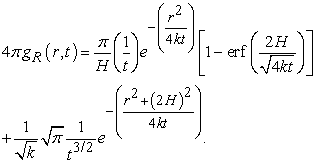(26)

Series Sum: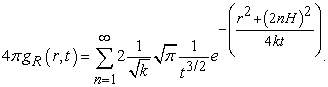(27)

These figures show the very good accuracy of the Euler-Maclaurin closed loop sum for a wide range H, r range which includes H=.3 to 4.5 m and r=.15 to 12 m.

### 4. Input Applications

The partial differential equation for acoustical diffusion is obtained by applying Eq. (2) for the energy density w(x,y,z,t).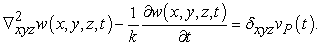(28)

where vp(t) is an input proportional to sound power and k has the units of m2/sec.

A point monopole source of surface area S, modeled as a pulsating sphere with a surface velocity u(t) produces a sound power P(t)=Sρc u2(t).

4.1. Transient Acoustic Energy Density Response to an Impact Input

Green’s function results in section 2 are directly applicable to a pure model of a sound impact with a surface velocity input u(t) =U δ(t) whereThus u2(t) ~ δ2(t) which is equivalent to u2(t) ~ δ(t) and vp(t) can be expressed as vp(t) =A δ(t). The units of A are power units/velocity squared units. The energy density is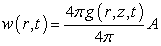where 4πg(r,z,t) is from Eq. (23) or Eq. (24) respectively for the Euler-Maclaurin closed form or the Infinite Series form. Units of w(r,z,t) are N/m2.

An exponential surface velocity input u(t) =umaxe-at represents an impact type of input that instantaneously rises and then decays to zero. Following the logic above, vp(t) becomes vp(t)=Be-αt where α=2a and B has the units of power units-sec/velocity squared and α is considered small, 0 ≤ α ≤1. Convolution in the time domain is given by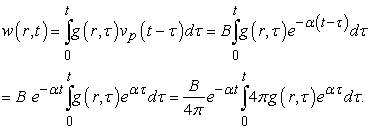(29)

Defining a relative energy density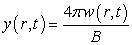and using the Green’s functions of Eq. (23) and Eq. (24),(30)(31)

Figure 6 is an example for α=.5 for typical cases of a close fitting/tight enclosure and an industrial space. The accuracy of the Euler-Maclaurin sum for the exponential input responses are similar to those of the Green’s function responses in Figure 4 and Figure 5.

4.2. Transient Thermal Heat Response to a Constant Input

The partial differential equation for heat diffusion is obtained by applying Eq. (2) for the Temperature T(x,y,z,t) in °K.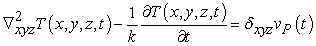(32)

where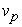(t)=B is a constant input proportional to heat flow and k has the units of m2/sec. B has the units of m-°K and can be expressed as B=μq where q is the input heat flow (watts/m2) and μ has the units of m-°K-sec3/kg.

Defining a relative temperatue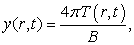Eq. (30) and Eq. (31) can be used by setting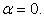Figure 7 is an example of a .15 m thick copper slab with k=.0012 and insulated at z=0 and z=H.

The accuracy of the Euler-Maclaurin closed form model is very good. The shape and trend of the curves in Figure 7 (a) are very similar to that calculated in 15 using a finite difference approach.

4.3. Frequency Response
4.3.1. Transfer Function

Taking the Laplace transform of the Green’s functions of Eq. (23) for the Euler-Maclaurin sum and Eq (24) (with z=zo=0) for the Series, the system transfer function for each of two models is obtained. From 12, the following exact transform pairs are available:

Defining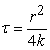and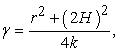the first and third term in 4πg(r,t)EM have the pairs:(33)

For the main integral term (the second term) in the Euler-Maclaurin sum, an exact Laplace transform does not exist, and an exponential approximation is used for the error function. Defining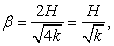consider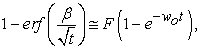where radian frequency parameter wo and amplitude factor F are determined from the minimization ofover a period of time. The Laplace transform of the second term in the Euler-Maclaurin sum is given by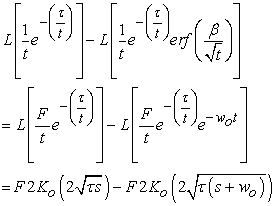(34)

Where Ko is the Bessel function of the second kind of order 0.

From Eq. (33) and Eq. (34), the transfer function for the Euler-Maclaurin sum is given by(35)

The transfer function for the series from Eq. (24) (with z=zo=0) is determined by using the forms in Eq. (31) and is given by(36)

whereFigure 8 gives a comparison between the Euler-Maclaurin transfer function of Eq. (35) and the Series transfer function Eq. (36) for the cases H=.3 m, r=.9 m., ωo=1, F=.4 and H=3 m, r=9 m, ωo=1, F=.8. The results are given across three mid-range octaves typical of acoustical broad band noise and indicate that the transfer function may be useful for controlling energy density in a diffuse acoustic environment.

• Figure 6. Exponential input example of Euler-Maclaurin closed form to Series form total solutions (direct + reverb) for α=.5. EM Eq. (30) (________) vs. Series Eq. (31) (- - - - - -). (a) H=.3 m, r=.9 m, (b) H=3 m., r=9 m
• Figure 7. Constant heat flow input example for slab (H=.15 ,. R=.03 m). Comparison of Euler-Maclaurin closed form to Series form of total solution (direct + reverb), including components. (a) EM Eq. (30 with α=0) (________) vs. Series Eq. (32 with α=0) (- - - - - -). (b) EM integral (________), ½ f(0) term (- - - - - -), 1st two terms (direct terms) in Eq. (30) (- . - . -)
• Figure 8. Transfer function example of Euler-Maclaurin closed form to Series form total solutions (direct + reverb). EM Eq. (35) (________) vs. Series Eq. (36) (- - - - - -). Row (a) H=.3 m, r=.9 m, Row (b) H=3 m., r=9 m

4.3.2. Feedback Control of Random Input

To test the use of the Euler-Maclaurin closed form model for reducing the response to a broad band noise source disturbanceFigure 9 gives the schematic for a model based feed forward control scheme that is combined with output feedback and a proportional amplification Kp of the error e=yd - y between the actual response y and a desired response yd. GS(s) represents the actual transfer function for the region. The series expression Eq. (36) for GS(s) is used here in simulations.

• Figure 9. Model-Based Control Scheme

The closed loop system output response is given by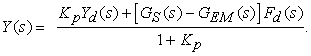(37)

To obtain a form that shows how the closed form Euler-Maclaurin model may reduce the system response for any desired input or disturbance, first divide by Yd(s).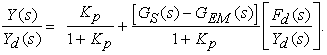(38)

Consider Kp large enough compared to 1 such that 1+ Kp can be replaced with Kp. Eq. (38) is reduced to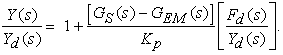(39)

If we define the parameter-1 and as a performance measure of the control scheme, a parameter Ф which is equal to the ratio of P with the feed forward part GEM(s) model to P without GEM(s), we get(40)
• Figure 10. Magnitude of control effectiveness measure |Ф(jω)|. (a) H=.3 m, r=.9 m, (b) H=3 m., r=9 m

The term group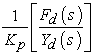cancels and Ф(s=jω) is given by(41)

In Eq. (41), if GEM(iω)= GS(iω), then Ф=0 which means a perfect match of model to actual system transfer functions will completely negate the disturbance and provide an almost perfect frequency trajectory match of Y(s) to the desired value Yd(s) when Kp is large enough relative to one. For smaller Kp, the factor Kp/(1+ Kp) comes into play. Figure 10 gives the magnitude of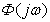from Eq. (41) for the cases H=.3 m, r=.9 m and H=3 m, r=9 m to show how the closed loop model can be effective is reducing the energy density when used in the control scheme presented. The results show a significant amount of attenuation over the three octave frequency bans typically associated with a broad band random noise input.

### 5. Conclusion

For an infinitely extending region bounded in a direction perpendicular to it and insulated such that no energy can flow through it, the work presented here shows that there is a connection between the Green’s function solutions to the acoustic partial differential equation wave equation and the partial differential equation for diffusion. The connection comes from extending the infinite series of images from the wave equation to the diffusion equation. The Euler-Maclaurin sum formula is applied to a modified form of images relevant for the diffusion equation to provide a closed loop solution to the diffusion equation for the region. Numerical examples comparing series and closed form representations are used to show the good accuracy of the closed form Green’s function for the response to transient inputs. Several cases of the acoustic diffusion partial differential equation representing reverberation in geometries ranging from tightfitting enclosures to industrial spaces are presented to show the accuracy of the closed form Euler-Maclaurin sum. A calculation for heat conduction in a thick slab with a concentrated constant heat source shows similar accuracy as related to infinite series solution vs. the Euler-Maclaurin closed form sum solution. Transfer functions obtained from the Green’s functions show good agreement between Series and Euler-Maclaurin models over a broad range of frequencies covering three octaves of mid-level frequencies that would be applicable to acoustic diffusion. Finally, a model based closed loop control scheme composed of both feedforward and feedback paths indicates that the use of the closed form Euler-Maclaurin model can be effective in reducing diffuse acoustic energy density in the region.

### References

  Panza M. J.,“Closed form solution for acoustic wave equation between two parallel plates using Euler-Maclaurin sum formula”, Journal of Sound and Vibration, 277, 123-132, 2004. In article View Article  Panza M. J., “Application of Euler–Maclaurin sum formula to obtain an approximate closed-form Green’s function for a two-dimensional acoustical space”, Journal of Sound and Vibration, 311, 269-279, 2008. In article View Article  Panza M. J., “Euler-Maclaurin closed form finite state space model for a string applied to broadband plate vibrations”, Journal of Mathematical Problems in Engineering, Vol. 2010, 2010. In article View Article  Panza M. J., “A finite state space model for representing the broadband infinite series for acoustic reverberation between parallel reflecting planes”, Journal of Mathematical Problems in Engineering, Vol.2018, 2018. In article View Article  Navarroa J. M. and Escolano J. “Simulation of building indoor acoustics using an acoustic diffusion equation model”, Journal of Building Performance Simulation, 8, 1-2, 2015. In article View Article  Hernández M., Imbernón, B., Navarro J. M., García, J. M. and Cebrian J. M., “Evaluation of the 3-D finite difference implementation of the acoustic diffusion equation model on massively parallel architectures”, Computers and Electrical Engineering, 46, 190-201, 2015. In article View Article  Gül Z. S., Xiang N. and Çalışkan M., “Sound field analysis of monumental structures by the application of diffusion equation model”, in Proceedings 2014 COMSOL, Cambridge Publishers. In article  Jing Y. and Xianga N., “A modified diffusion equation for room-acoustic predication”, Journal of the Acoustical Society of America, 121, 3284-3287, 2007. In article View Article  PubMed  Valeau V, Pacuat J. and Hodgson M., “On the use of a diffusion equation for room-acoustic prediction”, Journal of the Acoustical Society of America, 119, 1504-1513, 2006. In article View Article  Constanda C., Solution Techniques for Elementary Partial Differential Equations, Chapman & Hall/CRC Publishers, Boca Raton, FL, 207-217, 2010. In article  Hayek S. I., Advanced Mathematical Methods in Science and Engineering, Marcel Dekker Publishers, New York, 2001. In article  Oberhettinger F. and Badii L, Tables of Laplace Transforms, Springer-Verlag Publishers, New York, 1973, 41. In article View Article  Billon A., Picaut J., Valeau V. and Sakout A., “Acoustic Predictions in Industrial Spaces Using a Diffusion Model”, Advances in Acoustics and Vibration, Vol. 2012, 2012 In article View Article  Panza, M., “A Mathematical Images Group Model to Estimate the Sound Level in a Close-Fitting Enclosure”, Journal of Advances in Acoustics and Vibration, Vol. 2014, 2014. In article View Article  PubMed  Wu Z. C. and Cheng D. L, ”Temperature distribution of an infinite slab under point heat source”, Journal of Thermal Science, 18, 1597-1601, 2014. In article View ArticleThis work is licensed under a Creative Commons Attribution 4.0 International License. To view a copy of this license, visit http://creativecommons.org/licenses/by/4.0/